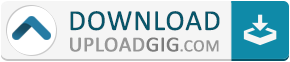#Do not remember me!

### Categories» » » Genetic Algorithm & Simulated Annealing in C++

## Genetic Algorithm & Simulated Annealing in C++Genetic Algorithm & Simulated Annealing in C++ | Size: 1.16 GB

Study these machine learning/optimization algorithms in continuous problems and the Travelling Salesperson Problem (TSP)
What you'll learn
The genetic algorithm & simulated annealing in C++
Genetic algorithm & simulated annealing on a continuous problem
Genetic algorithm on the travelling salesperson problem (TSP)
Requirements
Understand basic C++ and you should have a C++ IDE (any, I am using Visual Studio)
An understanding of some mathematics
An understanding of general algorithmics
An interest in cool algorithms ??
Description
This online course is for students and software developers who want to level up their skills by learning an interesting optimization algorithm in C++.
You will learn two of the most famous AI algorithms by writing it in C++ from scratch, so we will not use any libraries.
The Genetic Algorithm is the most famous one in a class called metaheuristics or optimization algorithms. You will learn what optimization algorithms are, when to use them, and then you will solve two problems with the Genetic Algorithm(GA). The second most famous one is Simulated Annealing.
These problems are: a continuous problem(find the maximum/minimum of a continuous function) and the Travelling Salesperson Problem (TSP), where you have to find the shortest path in a network of cities.
Prerequisites:
understand basic C++
any C++ IDE (I am using Visual Studio)
understanding of algorithms
understand mathematics
I recommend that you do the examples yourself, instead of passively watching the videos.
Here's a brief outline of what you will learn:
What optimization algorithms are
Genetic Algorithm theory:
General structure
How crossover is done
How mutation is done
Genetic Algorithm on a continuous problem:
Challenges particular to continuous problems: decoding the bits ("chromosomes") into a float value
Crossover: tournament selection and single point crossover
Mutation
Genetic Algorithm on the TSP (Travelling Salesperson Problem):
Creating a fitness function for the TSP
Challenge particular to this problem: how to do crossover?
Mutation
Simulated Annealing:
Basic Theory
Optimizing Himmelblau's function
Who this course is for:
Students and software developers who want to learn interesting algorithms
Anyone interested in this metaheuristic algorithmName:* E-Mail: Security Code: *